# Class 6 Maths NCERT Solutions for Chapter 7 Fractions Ex – 7.5

## Fractions

Question 1.
Write these fractions appropriately as additions or subtractions:Solution: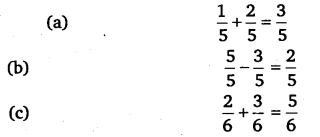Question 2.
Solve: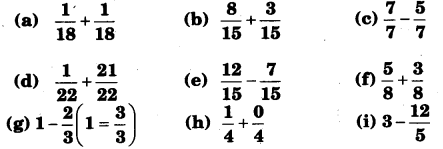Solution: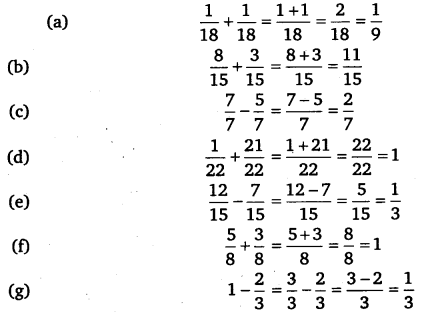Question 3.
Shubham painted$\frac { 2 }{ 3 }$ of the wall space in his room. His sister Madhavi helped and painted$\frac { 1 }{ 3 }$ of the wall space. How much did they paint together?

Solution:
Portion of wall painted by Shubham =$\frac { 2 }{ 3 }$
Portion of wall painted by Madhavi =$\frac { 1 }{ 3 }$
Wall painted by both of them =$\frac { 2 }{ 3 } +\frac { 1 }{ 3 } =\frac { 2+1 }{ 3 } =\frac { 3 }{ 3 } =1$
i.e., full wall was painted by them.

Question 4.
Fill in the missing fractions.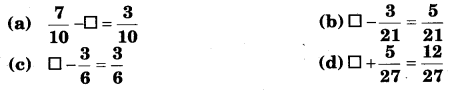Solution: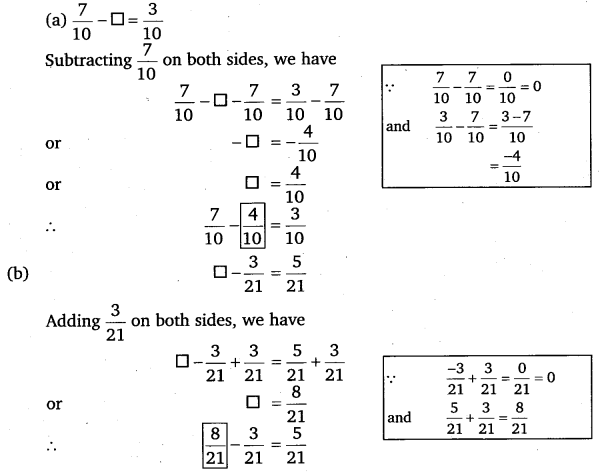Question 5.
Javed was given$\frac { 5 }{ 7 }$ of a basket of oranges. What fraction of oranges was left in the basket? ‘

Solution:
Portion of oranges received by Javed =$\frac { 5 }{ 7 }$
Portion of oranges left in the basket